# Fractions + direct relationship - math problems

#### Number of problems found: 70

• ExpressionIf it is true that ? is:
• SupermarketIn a local supermarket, 3/5 kilograms of squid cost 156.00. How do 4 kilograms of squid cost?
• Cyclist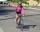Cyclist drives 5 km in half an hour, how long will drive in 3 hours?
• A bucketA bucket has 4 liters of water in it when it is 2/5 full. How much can it hold?
• Chicks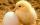How many chicks were hatched from 4500 eggs, when an average of 100 eggs hatched 87 chicks?
• Change the numbers in the ratio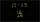Change the numbers 29, 38, and 43 in a 3: 4 ratio.
• Divide moneyDivide 1200 USD at a ratio of 1:2:3:4:5:6:9:10
• BuildingAt the building, we divided 240 boards into two piles in a 5: 3 ratio. How many were fewer boards in the lower pile?
• Tennis balls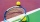Can of tennis balls contains 3 balls per can and cost \$7 how much will it cost for 36 tennis balls?
• Report 2A School reports students to teacher ratio of 6:1. If there are 45 teachers in the School, how many students are there?
• Grandmother's clocks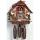Grandmother's clock is late every hour by half a minute. Grandmother set the clock exactly at 8.00 am. How many hours will show after 24 hours?
• Brass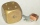Brass is an alloy of copper and zinc in a ratio of 3: 2. How many grams does a component that required 270 g of copper weigh?
• Photo egative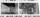Negative dimensions are 36mm and 28mm. What will be the photo size in the 21:4 ratio?
• StudyFor 7 hours of study, she scored a 75, so if she made 93, how many hours would she need to study?
• Kilo candyOne kilogram of chocolate candy cost 10 euros. Kate buys 250 grams and had the 8 pack of candy. How much does cost one bonbon?
• Composite ratioJakub, Aneta, and Lenka divided 1342 USD in the ratio 5/2: 3/10: 1/4. How much did Lenka take?
• TV failThe TV has after 10,000 hours average 35 failures. Determine the probability of TV failure after 400 hours of operation.
• BonusThe gross wage was 1323 USD including 25% bonus. How many USD were bonuses?
• DivideDivide area of rectangles with dimensions 32m and 10m by the ratio 7: 9. What area corresponds to a smaller section?
• Snack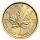CZK 450 was paid for a snack for 30 pupils. How many CZK will we pay for the same snack for 28 pupils?

Do you have an interesting mathematical word problem that you can't solve it? Submit a math problem, and we can try to solve it.

We will send a solution to your e-mail address. Solved examples are also published here. Please enter the e-mail correctly and check whether you don't have a full mailbox.

Please do not submit problems from current active competitions such as Mathematical Olympiad, correspondence seminars etc...
Need help to calculate sum, simplify or multiply fractions? Try our fraction calculator. Direct relationship - math problems. Fraction Word Problems.